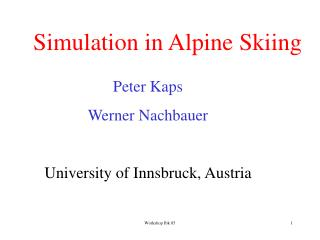DownloadDownload PresentationSimulation in Alpine Skiing

# Simulation in Alpine Skiing

Download Presentation## Simulation in Alpine Skiing

- - - - - - - - - - - - - - - - - - - - - - - - - - - E N D - - - - - - - - - - - - - - - - - - - - - - - - - - -
##### Presentation Transcript

1. Simulation in Alpine Skiing Peter Kaps Werner Nachbauer University of Innsbruck, Austria Workshop Ibk 05

2. Data Collection • Trajectory of body points • Landing movement after jumps in • Alpine downhill skiing, Lillehammer • (Carved turns, Lech) • Turn, World Cup race, Streif, Kitzbühel Workshop Ibk 05

3. Optimal landing Workshop Ibk 05

4. Landing in backward position Workshop Ibk 05

5. Workshop Ibk 05

6. Workshop Ibk 05

7. Direct linear transformation x,yimage coordinates X,Y,Zobject coordinates biDLT-parameters Z y Y X x Workshop Ibk 05

8. Control points at Russi jump Workshop Ibk 05

9. Camera position at Russi jump Workshop Ibk 05

10. Video frame on PC Workshop Ibk 05

11. Unconstrained Newton-Euler equation of motion (x,y,z)T Rigid body center of gravity: y=(x,y,z)T Workshop Ibk 05

12. Constrained equation of motion in 2D unconstrained r=0 Workshop Ibk 05

13. Constrained Newton-Euler equation of motion f applied forces r reaction forces geometric constraint d‘Alembert‘s principle DAE Workshop Ibk 05

14. Constrained Newton-Euler equation of motion DAE index 3 position level index 2 velocity level index 1 acceler. level Workshop Ibk 05

15. Equation of motion T(u,t)v Index-2-DAE Solved with RADAU 5 (Hairer-Wanner) MATLAB-version of Ch. Engstler Workshop Ibk 05

16. Jumps in Alpine skiing Ton van den Bogert Karin Gerritsen Kurt Schindelwig Workshop Ibk 05

17. Fz,Ende Fy,Ende Fz,Mitte Fy,Mitte Fz,Vorne Schneeoberfläche Skispitze Force between snow and ski Force between snow and ski normal to snow surface 3 nonlinear viscoelastic contact elements Workshop Ibk 05

18. Musculo-skeletal model of a skier muscle model van Soest, Bobbert 1993 Workshop Ibk 05

19. Muscle force production of force – contractile element ligaments - seriell elastic element connective tissue - parallel elastic element Workshop Ibk 05

20. LCE LPEE LSEE Muscle model of Hill total length L = LCE + LSEE CE contractile element SEE seriell elastic element PEEparallel elastic element Workshop Ibk 05

21. Force of seriell-elastic elements Force of parallel-elastic elements Workshop Ibk 05

22. Force-length-relation Fmax maximal isometric force isometric vCE= 0 maximal activation q = 1 Workshop Ibk 05

23. Force-velocity relation vCE = d/dt LCE maximal activation q = 1 optimal muscle length LCE = LCEopt Workshop Ibk 05

24. Hill equation (1938) Force-velocity relation concentric contraction Workshop Ibk 05

25. Activation model (Hatze 1981)muscle activation LCElength of the contractile elements calcium-ion concentration value of the non activated muscle Workshop Ibk 05

26. LCEopt optimal length of contractile elements Workshop Ibk 05

27. Activation model (Hatze 1981) Ordinary differential equation for the calcium-ion concentration  Control parameter: relative stimulation rate f stimulation rate, fmax maximum stimulation rate Workshop Ibk 05

28. LCE LPEE LSEE Equilibrium FCE(L,vCE,q) = f(L,LCE) Solving forvCE vCE = d/dt LCE = fH(L,LCE,q(,LCE)) Workshop Ibk 05

29. State of a muscle three state variables actual muscle length length of the contractile element calcium-ion conzentration Workshop Ibk 05

30. Force of muscle-ligament complex according to Hill-Modell Input: L, LCE,  compute equivalent torque muscle force times lever armDkfor jointk Dk constant Workshop Ibk 05

31. Comparison measured ( ) andsimulated ( ) landing movement Workshop Ibk 05

32. Turns in Alpine skiing Simulation with DADS Peter Lugner Franz Bruck Techn. University, Vienna Workshop Ibk 05

33. Trajectory of a ski racer x(t)=(X(t), Y(t), Z(t))T position as a function of time Mean value between the toe pieces of the left and right binding Track Position constraint Z-h(X,Y)=0 Y-s(X)=0 g(x,t)=0 Workshop Ibk 05

34. Equation of Motion Skier modelled as a mass point descriptor form dependent coordinates x Differential-Algebraic Equation DAE ODE algebraic equation f applied forces r reaction forces r = -gxT Workshop Ibk 05

35. Applied forces gravity snow friction drag t unit vector in tangential direction  friction coefficient N normal force N = ||r|| cd A drag area  density v velocity Workshop Ibk 05

36. Snow friction and drag area piecewice constant values determination of i , , ti by a least squares argument minimum x(ti) DAE-solution at time ti xi smoothed DLT-result at time ti Workshop Ibk 05

37. Software for Computation Computations were performed in MATLAB DAE-solver RADAU5 of Hairer-Wanner MATLAB-Version by Ch. Engstler Optimization problem Nelder-Mead simplex algorithmus Workshop Ibk 05

38. Results truncated values more exact values [0 , 0.1777] 1=0.4064 (cdA)1=0.9094 [0.1777, 0.5834] 2=0.4041 (cdA)2=0.9070 [0.5834, 1.9200] 1=0.1008 (cdA)1=0.5534 Workshop Ibk 05

39. Comparison more exact values Workshop Ibk 05

40. Comparison truncated values Workshop Ibk 05

41. Conclusions In Alpine skiing biomechanical studies under race conditions are possible. The results are reasonable, although circumstances for data collection are not optimal: no markers, position of control points must not disturb the racers, difficulties with commercial rights Results like loading of the anterior cruciate ligament (ACL) as function of velocity or inclination of the slope during landing or the possibility of a rupture of the ACL without falling are interesting applications in medicine. Informations on snow friction and drag in race conditions are interesting results, but a video analysis is expensive (digitizing the data, geodetic surveying). Workshop Ibk 05

42. Applications Determination of an optimal trajectory Virtual skiing, with vibration devices, in analogy to flight simulators Workshop Ibk 05